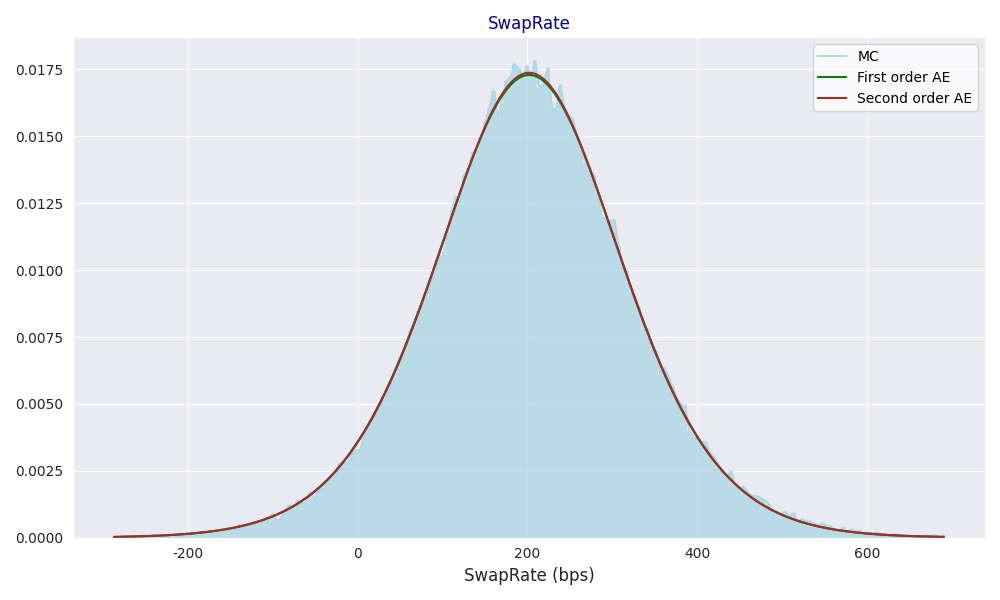• ## Robust Log-normal Stochastic Volatility for Interest Rate Dynamics – research paper

##### Posted at 5:49 pm by artursepp, on December 31, 2022

The volatility of interest rates in 2022 has been indeed extreme. In Figure 1, I show the dependence the between the MOVE index (which measures the implied volatility of one-month options on UST bond futures and which is constructed similarly to the VIX index for implied volatilities of the S&P index futures), realized 10y UST rate volatility over the 6 months rolling window, and the level of 10y UST rates. For understanding of historical patterns, we classify the historical period from 2002 to the end of 2022 into the 5 periods: 2002-2007 (hiking cycle), 2008-2010 (tightening), 2011-2017 (QE), 2018-2020 (tightening), 2021-2022 (hiking cycle).

We see that period of 2021-2022 was indeed unprecedented period when the rates rose from low levels of around 100 basis point (bp) to over 400bp, while the rates implied and realised volatilities rose from 50bps to over 150bps.Figure1. (A) The MOVE implied volatility index vs 10y UST bond rate; (B) 6m realized volatility of 10y UST bond rate vs 10y UST bond rate.

The dependence between the rate and its volatility manifests in implied volatilities with positive skews as I show in Figure 2 (The market convention is to use Bachelier normal model for marking implied swaption volatilities).Figure 2. Implied normal volatilities for $10Y$ swaption as function of option expiries in basis points observed in December 2022. Option delta is Bachelier normal model delta.

The dependence between the rate and volatility also manifests in strong level between the implied and realized volatilities and the volatility of volatility and the volatility beta (the change in 1bp of the volatility predicted by 1bp change in rates) which I show in Figure 3.Figure 3. (A) Realized volatility-of-volatility vs move volatility index. (B) Realized volatility beta vs Move index.

Quantitative modeling of such dynamics is challenging. In my previous joint paper with Parviz Rakhmonov on the
log-normal stochastic volatility for assets with positive return-volatility correlation we show that conventional SV model are ill-equipped for such dynamics. The rate dynamics are no exception, and practitioners rely on either local volatility models or local SV models with zero correlation. Both approaches are ill-poised because the may lead to explosive behavior of interest rates.

In our extension with Parviz we apply the log-normal SV dynamic for modelling the interest rate volatility, which is available on SSRN: Robust Log-normal Stochastic Volatility for Interest Rate Dynamics

We show that the proposed rates model is robust both on the quantitative dynamics and its practical implementation. While rate models in general are notorious for their tractability and implementation, we derive a closed form analytic solution for valuation of swaptions and for model calibration. In Figure 4, I show the model implied distribution of the 10y swap rate in the annuity measure computed using our analytical methods compared to the Monte Carlo simulations. Our solution is very accurate and it allows for robust calibration of the model to market data.Figure 4. Probability density functions computed using the first order affine expansion and the second-order expansion for the distribution of 10y swap rate in one year. The blue histogram is computed using realizations from MC simulations in model dynamics.

All the technical details are available in the paper: Robust Log-normal Stochastic Volatility for Interest Rate Dynamics. Happy reading.